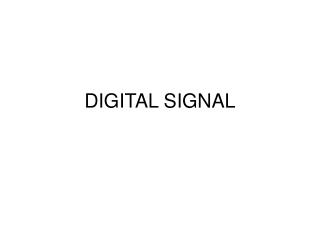DownloadDownload PresentationDIGITAL SIGNAL

# DIGITAL SIGNAL

Download Presentation## DIGITAL SIGNAL

- - - - - - - - - - - - - - - - - - - - - - - - - - - E N D - - - - - - - - - - - - - - - - - - - - - - - - - - -
##### Presentation Transcript

1. DIGITAL SIGNAL

2. Binary number

3. Binary number For decimal number 234.35= 2*102+ 2*101+4*100+3*10-1+5*10-2 For binary number 1101.01=1*23+1*22+0*21+1*20+0*2-1+1*2-2 That is 1101.01B=13.25D

4. Numeration System

5. reference voltage in “multiplying” DAC i.e., 00...0 => 0 volts; 11....1 => k volts (slightly less) k / 2n = “step size” Digital-to-analog conversion

6. Digital-to-analog conversion • When data is in binary form, the 0's and 1's may be of several forms such as the TTL form where the logic zero may be a value up to 0.8 volts and the 1 may be a voltage from 2 to 5 volts. • The data can be converted to clean digital form using gates which are designed to be on or off depending on the value of the incoming signal. Data in clean binary digital form can be converted to an analog form by using a summing amplifier. • For example, a simple 4 bit Dac can be made with a four-input summing amplifier. More practical is the R-2R Network DAC.

7. Digital-to-analog conversion One way to achieve D/A conversion is to use a summing amplifier